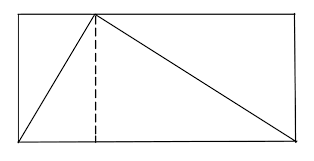Problems
328 - Simply Area

Simply Area

Time Limit: 1 sec

The Problem

If height is H and width is W

Then the area of a Rectangle is H * W

If we draw a Triangle into the Rectangle then

The Area of a Triangle is ½(rectangle area)

*The width is 4 times of heightThe Input

The input file contains a series of line. Each line contains a floating number Height, H (H <= 9999.99). Input is terminated by EOF.

The Output

For each line of input the output will show the Area of Rectangle rounded to 2 decimal points and ceiling points of Triangle.

Sample Input

1000.25

9965.23

Sample Output

Area of Rectangle: 4002000.25

Area of Triangle: 2001001

Area of Rectangle: 397223235.81

Area of Triangle: 198611618

Problem Setter: Samia Safa Ahmed